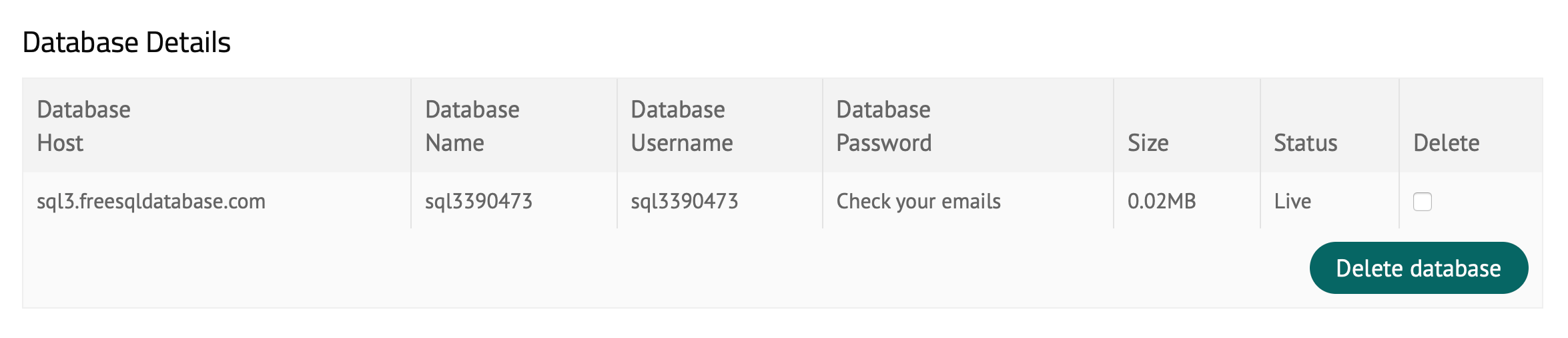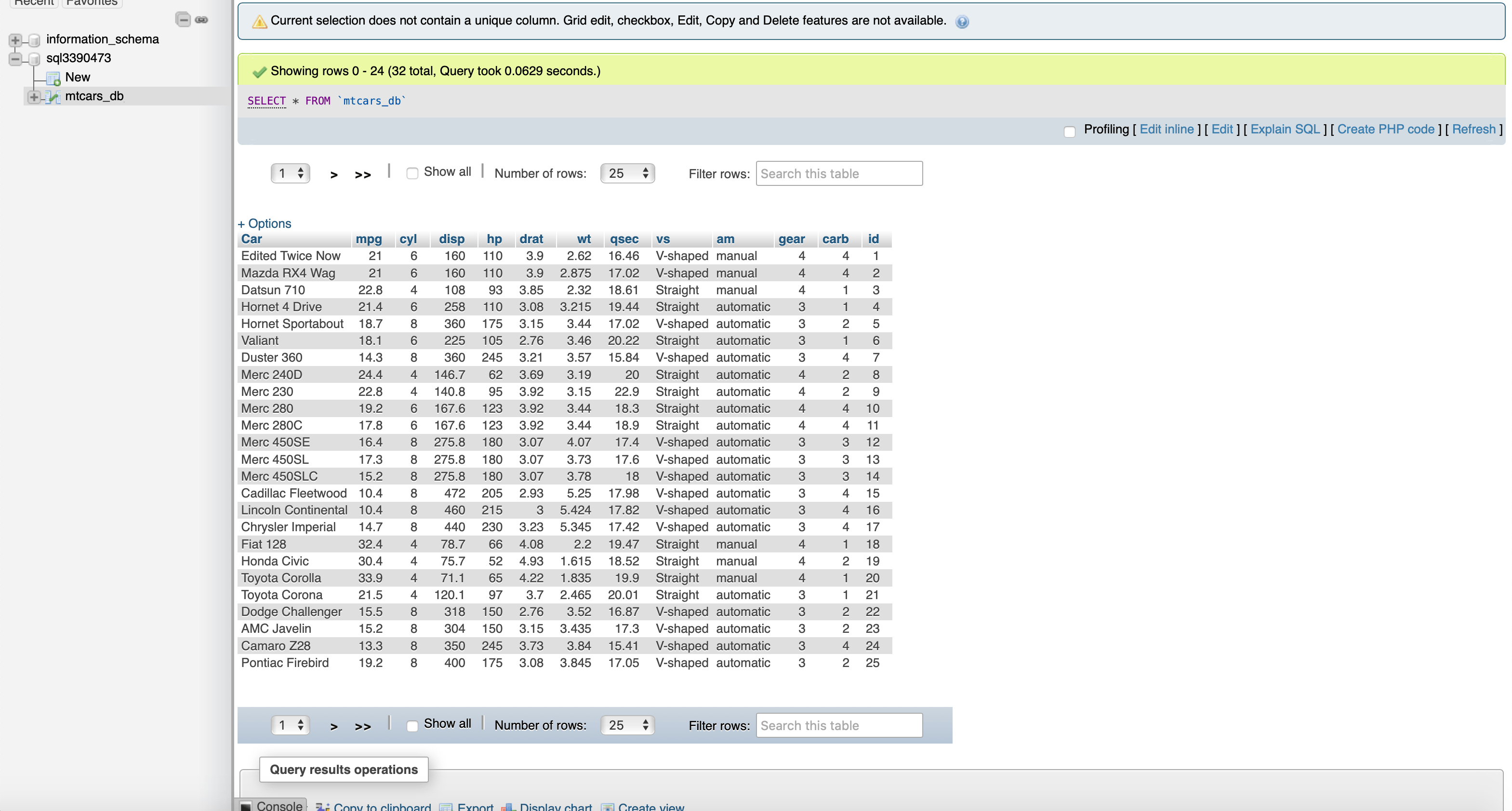Want to share your content on R-bloggers? click here if you have a blog, or here if you don't.

Last tutorial, we built a Shiny application where the user can add, delete, and edit specific row. Today we want to integrate a remote MySQL database for persistent data storage. When the user changes the anything of the table, the results are send to the MySQL database and will be loaded at the next session again.

If you want to check out the data table application from last time, you can find the blog post here.

We essentially only have to add some SQL queries in some observers and reactive functions where we add, delete, or edit the table.

Outline:

• Free remote MySQL database
• Database connection inside R
• Deleting rows in remote MySQL database
• Adding rows in remote MySQL database
• Editing rows in remote MySQL database

## Editing rows in remote MySQL database

You can sign up for a free remote database here. Connecting to the database is also super easy.There is the host, database name, username and password which you can find in your email. You can then connect to it inside R or can also inspect the the MySQL database.## MySQL Remote Database Connection for Shiny Application

We have stored the credentials to connect to the database in a `.yml` file.

```library(odbc)
library(RMySQL)
library(config)

config <- config::get(file = "datatable/config.yml")
con <- RMySQL::dbConnect(
RMySQL::MySQL(),
user = config\$db_user,
dbname = config\$db_name,
host = config\$db_host
)```

Now that we are connected, we get the data with:

```mtcars <- con %>%
dplyr::tbl("mtcars_db") %>%
dplyr::collect()```

and then we will be creating the action buttons which will allow the user to manipulate the table.

```create_btns <- function(x) {
x %>%
purrr::map_chr(~
paste0(
'<div class = "btn-group">
<button class="btn btn-default action-button btn-info action_button" id="edit_',
.x, '" type="button" onclick=get_id(this.id)><i class="fas fa-edit"></i></button>
<button class="btn btn-default action-button btn-danger action_button" id="delete_',
.x, '" type="button" onclick=get_id(this.id)><i class="fa fa-trash-alt"></i></button></div>'
))
}

x <- create_btns(mtcars\$id)
mtcars <- mtcars %>%
dplyr::bind_cols(tibble("Buttons" = x))```

You’ll notice that the action button ids are the same as the ids that are currently in the database id column. When we want to add, delete, and edit rows later, we will be identifying the rows in the database by getting the action button ids and comparing them to the id column in the database.

In order for the id column in the database to be unique, we are creating these reactive values:

```rv <- shiny::reactiveValues(
df = mtcars %>%
dplyr::select(-id),
dt_row = NULL,
edit_button = NULL,
keep_track_id = max(mtcars\$id) + 1
)```

where we delete the id column from the app because we do not want to show them in the application.

## Persistent Data Storage With a MySQL Database in R Shiny – Deleting Rows

The deleting of observers occurs in this observer:

```shiny::observeEvent(input\$current_id, {
shiny::req(!is.null(input\$current_id) &
stringr::str_detect(input\$current_id,
pattern = "delete"
))
rv\$dt_row <- which(stringr::str_detect(rv\$df\$Buttons,
pattern = paste0("\\b", input\$current_id, "\\b")
))

rv\$df <- rv\$df[-rv\$dt_row, ]
})```

Now, when we want to delete the row in the MySQL database as well, we have to add a `DELETE` query.

```### delete row
shiny::observeEvent(input\$current_id, {
shiny::req(!is.null(input\$current_id) &
stringr::str_detect(input\$current_id,
pattern = "delete"
))
rv\$dt_row <- which(stringr::str_detect(rv\$df\$Buttons,
pattern = paste0("\\b", input\$current_id, "\\b")
))

sql_id <- rv\$df[rv\$dt_row, ][["Buttons"]] %>%
stringr::str_extract_all(pattern = "delete_[0-9]+") %>%
unlist() %>%

query <- stringr::str_glue("DELETE FROM mtcars_db WHERE id = {sql_id}")
DBI::dbSendQuery(
con,
query
)

rv\$df <- rv\$df[-rv\$dt_row, ]
})```

In R, we deleted the row with the help of the which statement. We got the row we wanted to delete by getting the row of the action button. We cannot do the same with the database table. Hence, we get the row we want to delete by getting the unique action button id (which corresponds to the id column in our database) and then delete the row id = action button id. Lastly, we delete the row in the application as well.

## Persistent Data Storage With a MySQL Database in R Shiny – Adding Rows

```shiny::observeEvent(input\$final_edit, {
Car = input\$car_name,
mpg = input\$mpg,
cyl = input\$cyl,
disp = input\$disp,
hp = input\$hp,
drat = input\$drat,
wt = input\$wt,
qsec = input\$qsec,
vs = input\$vs,
am = input\$am,
gear = input\$gear,
carb = input\$carb,
Buttons = create_btns(rv\$keep_track_id)
)
dplyr::bind_rows(rv\$df)

dplyr::mutate(id = rv\$keep_track_id) %>%
dplyr::select(-Buttons)

column_names <- paste0(c(names(add_row)), collapse = ", ") %>%
paste0("(", ., ")")
values <- paste0("'", unlist(c(add_row)), "'", collapse = ", ") %>%
paste0("(", ., ")")
query <- paste0(
"INSERT INTO mtcars_db ",
column_names,
" VALUES ",
values
)
DBI::dbSendQuery(
con,
query
)

rv\$keep_track_id <- rv\$keep_track_id + 1
})```

The code above adds a row in our data table. The only thing we have to to is to add an id column to the row because an id column exists in our database. The we also have to deselect the action button column because we do not want to send the column to our database. Then we only have to write the query with the `INSERT` command and then send the query.

## Persistent Data Storage With a MySQL Database in R Shiny – Editing Rows

Editing rows is similar to adding rows.

```shiny::observeEvent(input\$final_edit, {
shiny::req(!is.null(input\$current_id) &
stringr::str_detect(input\$current_id, pattern = "edit") &

rv\$edited_row <- dplyr::tibble(
Car = input\$car_name,
mpg = input\$mpg,
cyl = input\$cyl,
disp = input\$disp,
hp = input\$hp,
drat = input\$drat,
wt = input\$wt,
qsec = input\$qsec,
vs = input\$vs,
am = input\$am,
gear = input\$gear,
carb = input\$carb,
Buttons = rv\$df\$Buttons[rv\$dt_row]
)

sql_row <- rv\$edited_row %>%
dplyr::select(-Buttons)

id <- rv\$df[rv\$dt_row, ][["Buttons"]] %>%
stringr::str_extract_all(pattern = "delete_[0-9]+") %>%
unlist() %>%

query <- paste0(
"UPDATE mtcars_db SET ",
paste0(names(sql_row), "=", "'", unlist(c(sql_row)), "'", collapse = ", "),
stringr::str_glue("WHERE id = {id}")
)
DBI::dbSendQuery(
con,
query
)

rv\$df[rv\$dt_row, ] <- rv\$edited_row
})```

We also deselect the buttons and then `UPDATE` the database table where the action button id in the application table is equal to the id in the database column.

Every time you close the app now and restart it again, it will load the data from the database and will store the changes you made.

## Entire R Shiny Application with Persistent Data Storage

```library(shiny)
library(tidyverse)
library(DT)
library(RMySQL)
library(config)
library(odbc)

source("modal_dialog.R")

config <- config::get(file = "datatable/config.yml")
con <- RMySQL::dbConnect(
RMySQL::MySQL(),
user = config\$db_user,
dbname = config\$db_name,
host = config\$db_host
)

mtcars <- con %>%
dplyr::tbl("mtcars_db") %>%
dplyr::collect()

create_btns <- function(x) {
x %>%
purrr::map_chr(~
paste0(
'<div class = "btn-group">
<button class="btn btn-default action-button btn-info action_button" id="edit_',
.x, '" type="button" onclick=get_id(this.id)><i class="fas fa-edit"></i></button>
<button class="btn btn-default action-button btn-danger action_button" id="delete_',
.x, '" type="button" onclick=get_id(this.id)><i class="fa fa-trash-alt"></i></button></div>'
))
}

x <- create_btns(mtcars\$id)
mtcars <- mtcars %>%
dplyr::bind_cols(tibble("Buttons" = x))

ui <- fluidPage(

# div(style = "display: none;", icon("refresh")),
div(
class = "container",
div(
style = "margin-top: 50px;",
shiny::actionButton(
icon = shiny::icon("plus"),
class = "btn-success"
)
)
),
div(
class = "container",
style = "margin-top: 50px;",
DT::DTOutput(outputId = "dt_table", width = "100%")
),

shiny::includeScript("script.js")
)

# Define server logic required to draw a histogram
server <- function(input, output, session) {
rv <- shiny::reactiveValues(
df = mtcars %>%
dplyr::select(-id),
dt_row = NULL,
edit_button = NULL,
keep_track_id = max(mtcars\$id) + 1
)

output\$dt_table <- DT::renderDT(
{
shiny::isolate(rv\$df)
},
escape = F,
rownames = FALSE,
options = list(processing = FALSE)
)

proxy <- DT::dataTableProxy("dt_table")
shiny::observe({
DT::replaceData(proxy, rv\$df, resetPaging = FALSE, rownames = FALSE)
})

### delete row
shiny::observeEvent(input\$current_id, {
shiny::req(!is.null(input\$current_id) &
stringr::str_detect(input\$current_id,
pattern = "delete"
))
rv\$dt_row <- which(stringr::str_detect(rv\$df\$Buttons,
pattern = paste0("\\b", input\$current_id, "\\b")
))

sql_id <- rv\$df[rv\$dt_row, ][["Buttons"]] %>%
stringr::str_extract_all(pattern = "delete_[0-9]+") %>%
unlist() %>%

query <- stringr::str_glue("DELETE FROM mtcars_db WHERE id = {sql_id}")
DBI::dbSendQuery(
con,
query
)

rv\$df <- rv\$df[-rv\$dt_row, ]
})

# when edit button is clicked, modal dialog shows current editable row filled out
shiny::observeEvent(input\$current_id, {
shiny::req(!is.null(input\$current_id) &
stringr::str_detect(input\$current_id,
pattern = "edit"
))
rv\$dt_row <- which(stringr::str_detect(rv\$df\$Buttons,
pattern = paste0("\\b", input\$current_id, "\\b")
))
df <- rv\$df[rv\$dt_row, ]
modal_dialog(
car = df\$Car, mpg = df\$mpg, cyl = df\$cyl,
hp = df\$hp, disp = df\$disp, drat = df\$drat,
wt = df\$wt, qsec = df\$qsec, vs = mtcars\$vs,
am = mtcars\$am, gear = df\$gear, carb = df\$carb,
selected_am = df\$am, selected_vs = df\$vs, edit = TRUE
)
})

# when final edit button is clicked, table will be changed
shiny::observeEvent(input\$final_edit, {
shiny::req(!is.null(input\$current_id) &
stringr::str_detect(input\$current_id, pattern = "edit") &

rv\$edited_row <- dplyr::tibble(
Car = input\$car_name,
mpg = input\$mpg,
cyl = input\$cyl,
disp = input\$disp,
hp = input\$hp,
drat = input\$drat,
wt = input\$wt,
qsec = input\$qsec,
vs = input\$vs,
am = input\$am,
gear = input\$gear,
carb = input\$carb,
Buttons = rv\$df\$Buttons[rv\$dt_row]
)

sql_row <- rv\$edited_row %>%
dplyr::select(-Buttons)

id <- rv\$df[rv\$dt_row, ][["Buttons"]] %>%
stringr::str_extract_all(pattern = "delete_[0-9]+") %>%
unlist() %>%

query <- paste0(
"UPDATE mtcars_db SET ",
paste0(names(sql_row), "=", "'", unlist(c(sql_row)), "'", collapse = ", "),
stringr::str_glue("WHERE id = {id}")
)
DBI::dbSendQuery(
con,
query
)

rv\$df[rv\$dt_row, ] <- rv\$edited_row
})

modal_dialog(
car = "", mpg = "", cyl = "", hp = "", disp = "", drat = "",
wt = "", qsec = "", vs = mtcars\$vs, am = mtcars\$am, gear = "", carb = "",
selected_am = NULL, selected_vs = NULL, edit = FALSE
)
})

shiny::observeEvent(input\$final_edit, {
Car = input\$car_name,
mpg = input\$mpg,
cyl = input\$cyl,
disp = input\$disp,
hp = input\$hp,
drat = input\$drat,
wt = input\$wt,
qsec = input\$qsec,
vs = input\$vs,
am = input\$am,
gear = input\$gear,
carb = input\$carb,
Buttons = create_btns(rv\$keep_track_id)
)
dplyr::bind_rows(rv\$df)

dplyr::mutate(id = rv\$keep_track_id) %>%
dplyr::select(-Buttons)

column_names <- paste0(c(names(add_row)), collapse = ", ") %>%
paste0("(", ., ")")
values <- paste0("'", unlist(c(add_row)), "'", collapse = ", ") %>%
paste0("(", ., ")")
query <- paste0(
"INSERT INTO mtcars_db ",
column_names,
" VALUES ",
values
)
DBI::dbSendQuery(
con,
query
)

rv\$keep_track_id <- rv\$keep_track_id + 1
})

### remove edit modal when close button is clicked or submit button
shiny::observeEvent(input\$dismiss_modal, {
shiny::removeModal()
})
shiny::observeEvent(input\$final_edit, {
shiny::removeModal()
})
}

onStop(function() {
dbDisconnect(con)
})

# Run the application
shinyApp(ui = ui, server = server)
```

Let me know if you have any questions in the comments below.

The post Persistent Data Storage With a MySQL Database in R Shiny – An Example App appeared first on .